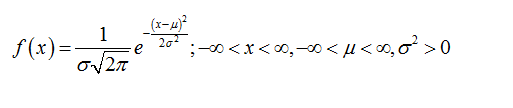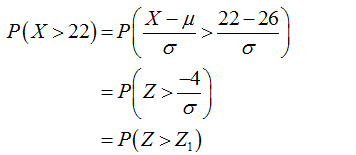# It is known that the length of a certain product X is normally distributed with U=26 inches. How is the Probability P(X>22) related to P(X<22)? Mulitple choice:1. P(X>22) is greater than P(X<22)2. P(X>22) is smaller than P(X<22)3. No comparison can be made without knowing the value of the standard deviation O

Question
4 views

It is known that the length of a certain product X is normally distributed with U=26 inches. How is the Probability P(X>22) related to P(X<22)?

Mulitple choice:

1. P(X>22) is greater than P(X<22)

2. P(X>22) is smaller than P(X<22)

3. No comparison can be made without knowing the value of the standard deviation O

check_circle

Step 1

The normal distribution:

A continuous random variable X is said to follow normal distribution with mean µ and standard deviation σ if the probability density function of X is,Step 2

It is given that X follows a normal distribution with µ =26.

The probability that   X greater than 22 is calculated as follows:...

### Want to see the full answer?

See Solution

#### Want to see this answer and more?

Solutions are written by subject experts who are available 24/7. Questions are typically answered within 1 hour.*

See Solution
*Response times may vary by subject and question.
Tagged in